• in the last video, we are addressing Recommender Systems problem that have set of movies and set of users and the problem is try to recommend movies they never seen, how they would rate it
• One approach to handle this is called Content-based Recommendation, which would discussed in this video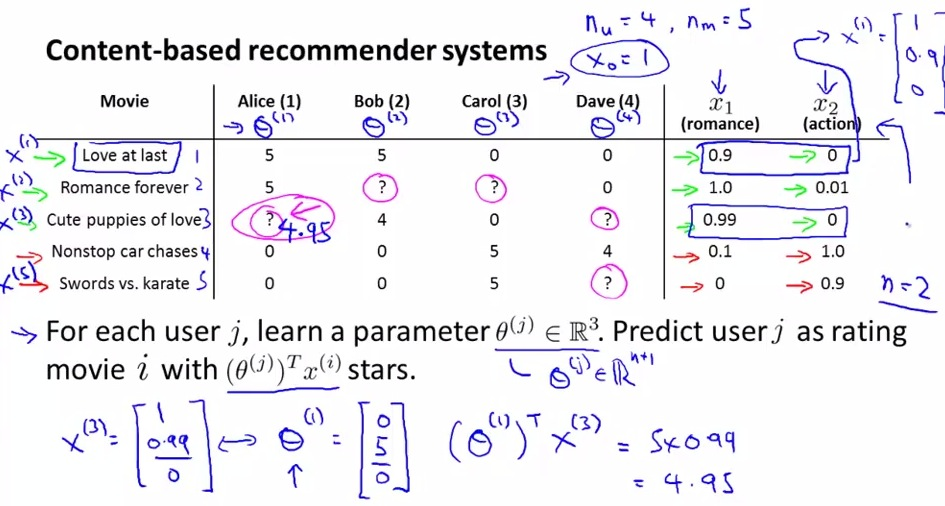• For the sake of the examples, we choose 2 features, as above, (will discussed later on how to choose features)
• the ratio of rating for each feature of the movies will vary between 0 to 1.
• Each feature will have feature vector, where x0 = 1 (inceptor)
• Theta is the parameter rated category of each user, which later would be discussed
• Theta is like how's one category is rated by user. x1 for user1 is 0.99, so she would rate movies in category x1 as 4.95
• So is similar as linear regression problem and how we apply them
• each feature is calculated on how much user would rate it, 5 rate = 1 in x.
• And so each user would have different guessed rating. Each theta is evaluated on how particular user rate the categories/features
• The value calculated on formula above(5x0.99 = 4.95) seems like a valid calculation
• theta0 = 0(not counting x0),
• theta1 = x1(rating  user would give on romance),t
• theta2 = x2(rating user would give on action)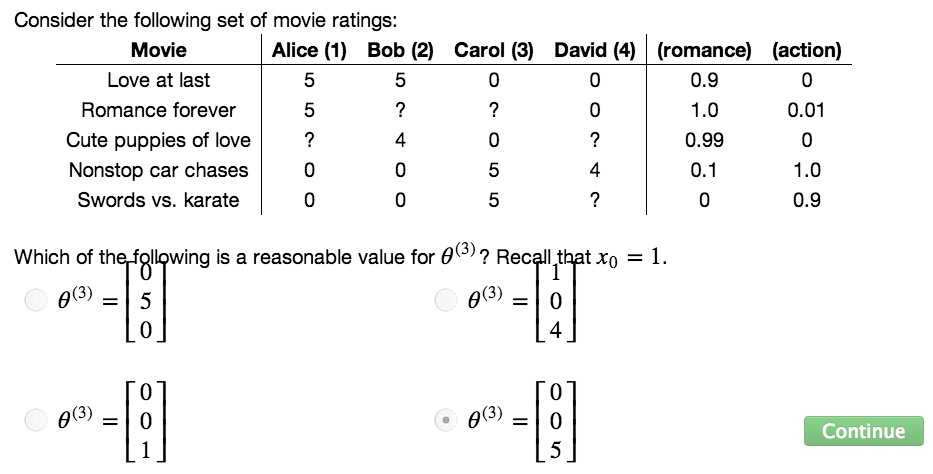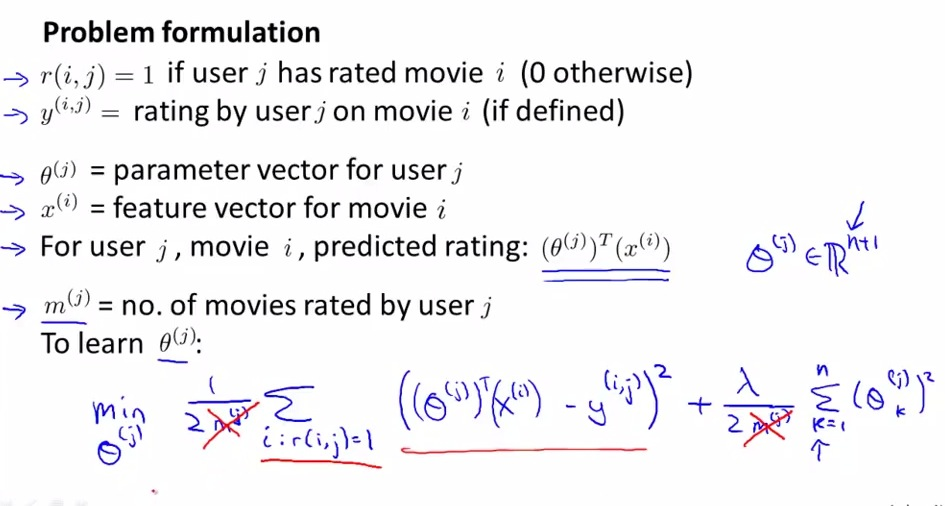• choose theta that as close as possible in training set
• Summation above means all the movies that user j has rated
• Similar as linear regression problem, except without mj
• n = number of features in movies
• The formula would give algorithm that predict the movies rated
• eliminate m(j) would still give same value theta j
• theta j would be n +1 dimensional vector, because we have additional 8theta0 = 0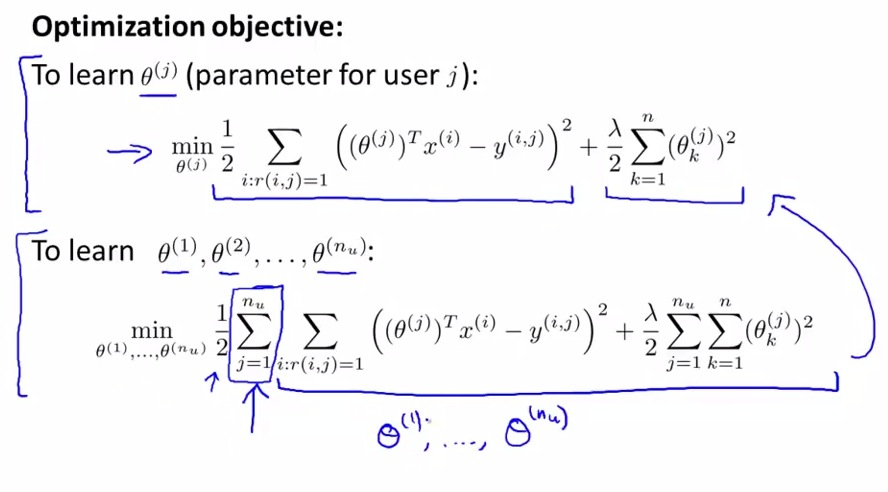• By now, we already have the formula without mj
• So given each user, we also may want to know how much the parameters prediction for all users, and try to minimize he cost function
• We also have different prediction for making prediction of all users rating.
• What explained above, is we just added the summation for all users in the cost function as well as in the regularization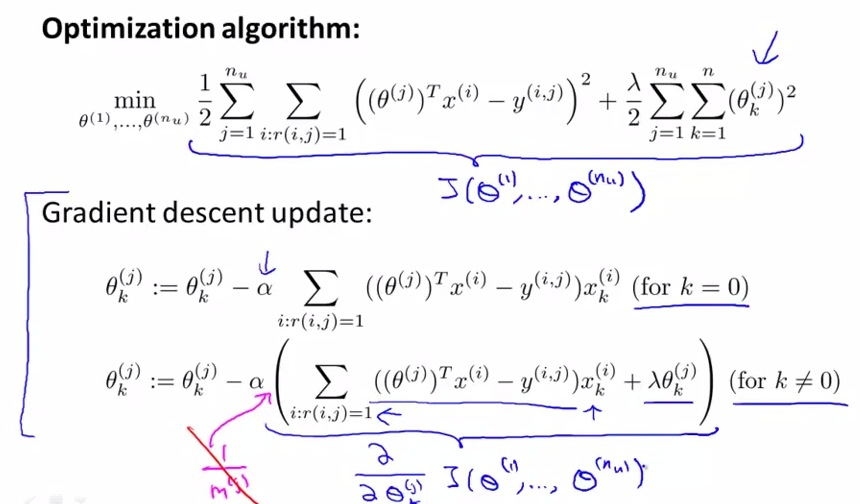• For Gradient descent update, depending of whether k = 0, we also have different formula
• in blue gathered is the partial derrivation, and use it to minimize cost  function in Gradient Descent
• Also can use other advanced optimization to try to minimum cost function J as swell

SUMMARY
• CBR is one approach for Recommender Systems problem, capture the content of the various movies, based on rating.
• Sometimes we face diffcult problems, that is in movie example, how's the movie that doesn't have clear rating/category.
• Next, how Recommender System face unrating product, that is worked for all product for all the content